# 算法流程

$\left|r_{ij}\right|^2-d_{ij}^2=0$

$r_{ij}\cdot v_{ij}=0$

## 约束前后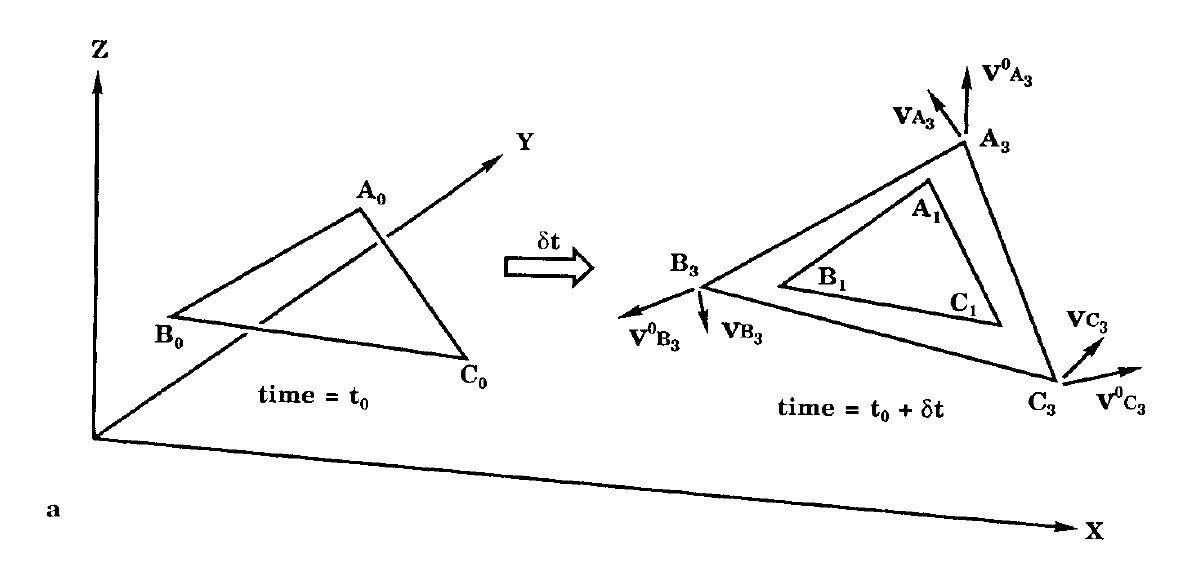## 约束算法中的约束条件## 建立新的坐标系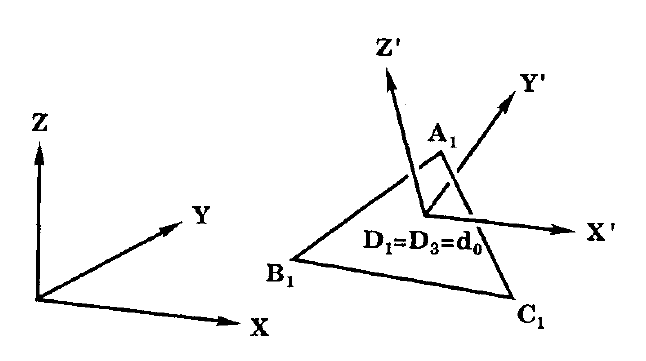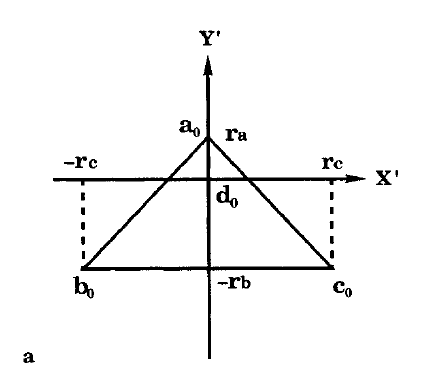## 三维旋转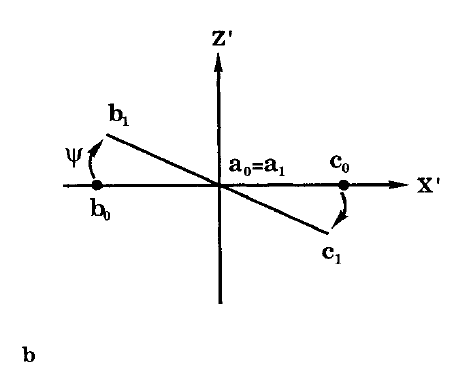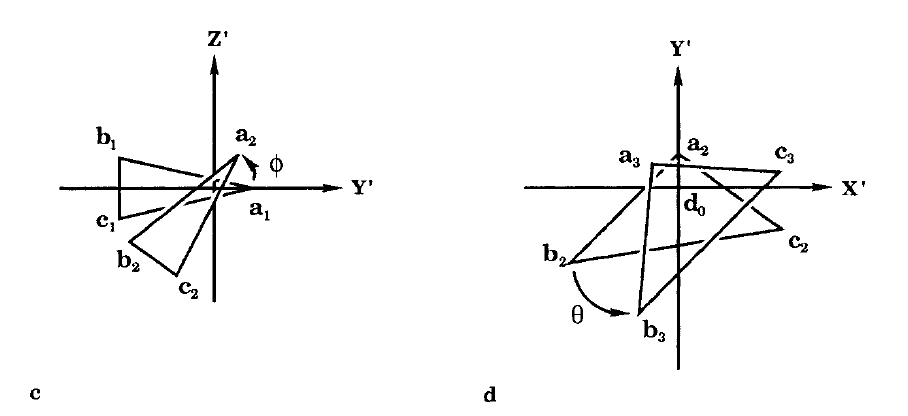# 算法解析表达式

\begin{align} a'_0&=[0,r_a,0]\\ b'_0&=[-r_c,-r_b,0]\\ c'_0&=[r_c,-r_b,0] \end{align}\begin{align} \phi&=arcsin\left(\frac{Z'_{A_1}}{r_a}\right)\\ \psi&=arcsin\left(\frac{Z'_{B_1}-Z'_{C_1}}{2r_ccos\phi}\right)\\ \theta&=arcsin\left(\frac{\gamma}{\sqrt{\alpha^2+\beta^2}}\right)-arctan\left(\frac{\beta}{\alpha}\right) \end{align}

\begin{align} \alpha&=-r_ccos\psi(X'_{B_0}-X'_{C_0})+(-r_bcos\phi-r_csin\psi sin\phi)(Y'_{B_0}-Y'_{A_0})+(-r_bcos\phi+r_csin\psi sin\phi)(Y'_{C_0}-Y'_{A_0})\\ \beta&=-r_ccos\psi(Y'_{C_0}-Y'_{B_0})+(-r_bcos\phi-r_csin\psi sin\phi)(X'_{B_0}-X'_{A_0})+(-r_bcos\phi+r_csin\psi sin\phi)(X'_{C_0}-X'_{A_0})\\ \gamma&=Y'_{B_1}(X'_{B_0}-X'_{A_0})-X'_{B_1}(Y'_{B_0}-Y'_{A_0})+Y'_{C_1}(X'_{C_0}-X'_{A_0})-X'_{C_1}(Y'_{C_0}-Y'_{A_0}) \end{align}

\begin{align} a'_3&=\left(-r_acos\phi sin\theta,\ r_acos\phi cos\theta,\ r_asin\phi\right)\\ b'_3&=\left(-r_ccos\psi cos\theta+r_bsin\theta cos\phi+r_csin\theta sin\psi sin\phi,\\ -r_ccos\psi sin\theta-r_bcos\theta cos\phi-r_ccos\theta sin\psi sin\phi,\\ r_csin\psi cos\phi\right)\\ c'_3&=\left(r_ccos\psi cos\theta+r_bsin\theta cos\phi-r_csin\theta sin\psi sin\phi,\\ r_ccos\psi sin\theta-r_bcos\theta cos\phi+r_ccos\theta sin\psi sin\phi,\\ -r_bsin\phi-r_csin\psi cos\phi \right) \end{align}

# 三维空间坐标变换

$R_Y(\psi)=\left( \begin{matrix} cos\psi && 0 && -sin\psi\\ 0 && 1 && 0\\ sin\psi && 0 && cos\psi \end{matrix} \right)\\ R_X(\phi)=\left( \begin{matrix} 1 && 0 && 0\\ 0 && cos\phi && -sin\phi\\ 0 && sin\phi && cos\phi \end{matrix} \right)\\ R_Z(\theta)=\left( \begin{matrix} cos\theta && -sin\theta && 0\\ sin\theta && cos\theta && 0\\ 0 && 0 && 1 \end{matrix} \right)$# 坐标变换代码实现

1. 通过python代码构建一个等腰三角形，通过旋转使得其达到一个初始位置$$A_0B_0C_0$$，这个初始位置对应上述章节中的三角形$$A_0B_0C_0$$，之所以要通过三个角度的旋转来实现，是为了同时演示这个三维旋转的过程，对应的是如下代码中的rotation函数；
2. 计算三角形$$A_0B_0C_0$$的质心center of mass，表示为$$M$$
3. 因为三个点就可以固定一个平面，这里我们选取的是$$A$$$$B$$这两个点以及$$\vec{BC}\otimes \vec{CA}$$这个向量（不能只取三角形所在平面上的点，否则无法正确求解方程，因为对应的参数矩阵秩为2），再假定一个旋转矩阵$$R$$，联立一个九元一次方程组，就可以解得旋转矩阵的具体值。\begin{align} R\left[\left(\begin{matrix} X_{A_0}\\ Y_{A_0}\\ Z_{A_0} \end{matrix}\right)-\vec{M}\right] &=\left(\begin{matrix} 0\\ r_a\\ 0 \end{matrix}\right)\\ R\left[\left(\begin{matrix} X_{B_0}\\ Y_{B_0}\\ Z_{B_0} \end{matrix}\right)-\vec{M}\right] &=\left(\begin{matrix} -r_c\\ -r_b\\ 0 \end{matrix}\right)\\ R\left[\begin{matrix} \vec{BC}\otimes\vec{CA} \end{matrix}\right] &=\left(\begin{matrix} 0\\ 0\\ 1 \end{matrix}\right) \end{align}

# settle.py
from jax import numpy as np
from jax import vmap, jit

def rotation(psi,phi,theta,v):
""" Module of rotation in 3 Euler angles. """
RY = np.array([[np.cos(psi),0,-np.sin(psi)],
[0, 1, 0],
[np.sin(psi),0,np.cos(psi)]])
RX = np.array([[1,0,0],
[0,np.cos(phi),-np.sin(phi)],
[0,np.sin(phi),np.cos(phi)]])
RZ = np.array([[np.cos(theta),-np.sin(theta),0],
[np.sin(theta),np.cos(theta),0],
[0,0,1]])
return np.dot(RZ,np.dot(RX,np.dot(RY,v)))

multi_rotation = jit(vmap(rotation,(None,None,None,0)))

if __name__ == '__main__':
import matplotlib.pyplot as plt
# construct params
ra = 0.5
rb = 0.7
rc = 1.2
psi = 0.4
phi = 0.5
theta = 1.3
# construct initial crd
crd = np.array([[0, ra, 0],
[-rc, -rb, 0],
[rc, -rb, 0]])
shift = np.array([0.1, 0.1, 0.1])
crd = multi_rotation(psi,phi,theta,crd) + shift
# get the center of mass
com = np.average(crd,0)
# 3 points are selected to solve the initial rotation matrix
xyz = [0,0,0]
xyz = crd-com
xyz = crd-com
cross = np.cross(crd-crd,crd-crd)
cross /= np.linalg.norm(cross)
xyz = cross
xyz = np.array(xyz)
inv_xyz = np.linalg.inv(xyz)
v0 = np.array([0,-rc,0])
v1 = np.array([ra,-rb,0])
v2 = np.array([0,0,1])
# final rotation matrix is constructed by following
Rot = np.array([np.dot(inv_xyz,v0),np.dot(inv_xyz,v1),np.dot(inv_xyz,v2)])
print (Rot)
# some test cases and results
origin = crd
print(np.dot(Rot, origin-com))
# [1.4901161e-08 5.0000000e-01 0.0000000e+00]
origin = crd
print(np.dot(Rot, origin-com))
# [-1.2000000e+00 -7.0000005e-01 -5.9604645e-08]
origin = crd
print(np.dot(Rot, origin-com))
# [ 1.2000000e+00  2.0000000e-01 -1.4901161e-08]
origin = xyz
print(np.dot(Rot, origin))
# [0.0000000e+00 2.9802322e-08 1.0000000e+00]# SETTLE代码实现

1. 获取$$XYZ$$坐标系下三角形$$A_0B_0C_0$$的坐标以及三角形$$A_1B_1C_1$$的坐标；
2. 根据三角形$$A_0B_0C_0$$的坐标计算得$$r_a,r_b,r_c$$的值以及质心$$M$$的坐标；
3. 根据三角形$$A_0B_0C_0$$的坐标以及$$r_a,r_b,r_c$$的值和质心$$M$$的坐标，计算$$XYZ$$坐标系到$$X'Y'Z'$$坐标系的变换矩阵$$Rot$$及其逆变换$$Rot^{-1}$$
4. $$Rot$$和质心坐标计算三角形$$A_1B_1C_1$$在坐标系$$X'Y'Z'$$下的坐标；
5. 根据三角形$$A_1B_1C_1$$在坐标系$$X'Y'Z'$$下的坐标计算得三个旋转角$$\phi,\psi,\theta$$
6. 根据$$\phi,\psi,\theta$$$$r_a,r_b,r_c$$计算三角形$$A_3B_3C_3$$，也就是我们的目标三角形，在$$X'Y'Z'$$坐标系下的坐标；
7. 使用$$Rot^{-1}$$将三角形$$A_3B_3C_3$$在坐标系$$X'Y'Z'$$下的坐标变换回坐标系$$XYZ$$下的坐标，至此就完成了SETTLE算法的实现。

# settle.py
from jax import numpy as np
from jax import vmap, jit

def rotation(psi,phi,theta,v):
""" Module of rotation in 3 Euler angles. """
RY = np.array([[np.cos(psi),0,-np.sin(psi)],
[0, 1, 0],
[np.sin(psi),0,np.cos(psi)]])
RX = np.array([[1,0,0],
[0,np.cos(phi),-np.sin(phi)],
[0,np.sin(phi),np.cos(phi)]])
RZ = np.array([[np.cos(theta),-np.sin(theta),0],
[np.sin(theta),np.cos(theta),0],
[0,0,1]])
return np.dot(RZ,np.dot(RX,np.dot(RY,v)))

multi_rotation = jit(vmap(rotation,(None,None,None,0)))

def get_rot(crd):
""" Get the coordinates transform matrix. """
# get the center of mass
com = np.average(crd, 0)
rc = np.linalg.norm(crd-crd)/2
ra = np.linalg.norm(crd-com)
rb = np.sqrt(np.linalg.norm(crd-crd)**2-rc**2)-ra
# 3 points are selected to solve the initial rotation matrix
xyz = [0, 0, 0]
xyz = crd - com
xyz = crd - com
cross = np.cross(crd - crd, crd - crd)
cross /= np.linalg.norm(cross)
xyz = cross
xyz = np.array(xyz)
inv_xyz = np.linalg.inv(xyz)
v0 = np.array([0, -rc, 0])
v1 = np.array([ra, -rb, 0])
v2 = np.array([0, 0, 1])
# final rotation matrix is constructed by following
Rot = np.array([np.dot(inv_xyz, v0), np.dot(inv_xyz, v1), np.dot(inv_xyz, v2)])
inv_Rot = np.linalg.inv(Rot)
return Rot, inv_Rot

def xyzto(Rot, crd, com):
""" Apply the coordinates transform matrix. """
return np.dot(Rot, crd-com)

multi_xyzto = jit(vmap(xyzto,(None,0,None)))

def toxyz(Rot, crd, com):
""" Apply the inverse of transform matrix. """
return np.dot(Rot, crd-com)

multi_toxyz = jit(vmap(toxyz,(None,0,None)))

def get_circumference(crd):
""" Get the circumference of all triangles. """
return np.linalg.norm(crd-crd)+np.linalg.norm(crd-crd)+np.linalg.norm(crd-crd)

jit_get_circumference = jit(get_circumference)

def get_angles(crd_0, crd_t0, crd_t1):
""" Get the rotation angle psi, phi and theta. """
com = np.average(crd_0, 0)
rc = np.linalg.norm(crd_0 - crd_0) / 2
ra = np.linalg.norm(crd_0 - com)
rb = np.sqrt(np.linalg.norm(crd_0 - crd_0) ** 2 - rc ** 2) - ra
phi = np.arcsin(crd_t1/ra)
psi = np.arcsin((crd_t1-crd_t1)/2/rc/np.cos(phi))
alpha = -rc*np.cos(psi)*(crd_t0-crd_t0)+(-rb*np.cos(phi)-rc*np.sin(psi)*np.sin(phi))*(crd_t0-crd_t0)+ \
(-rb*np.cos(phi)+rc*np.sin(psi)*np.sin(phi))*(crd_t0-crd_t0)
beta = -rc*np.cos(psi)*(crd_t0-crd_t0)+(-rb*np.cos(phi)-rc*np.sin(psi)*np.sin(phi))*(crd_t0-crd_t0)+ \
(-rb*np.cos(phi)+rc*np.sin(psi)*np.sin(phi))*(crd_t0-crd_t0)
gamma = crd_t1*(crd_t0-crd_t0)-crd_t1*(crd_t0-crd_t0)+\
crd_t1*(crd_t0-crd_t0)-crd_t1*(crd_t0-crd_t0)
sin_part = gamma/np.sqrt(alpha**2+beta**2)
theta = np.arcsin(sin_part)-np.arctan(beta/alpha)
return phi, psi, theta

jit_get_angles = jit(get_angles)

def get_d3(crd_0, psi, phi, theta):
""" Calculate the new coordinates by 3 given angles. """
com = np.average(crd_0, 0)
rc = np.linalg.norm(crd_0 - crd_0) / 2
ra = np.linalg.norm(crd_0 - com)
rb = np.sqrt(np.linalg.norm(crd_0 - crd_0) ** 2 - rc ** 2) - ra
return np.array([[-ra*np.cos(phi)*np.sin(theta), ra*np.cos(phi)*np.cos(theta), ra*np.sin(phi)],
[-rc*np.cos(psi)*np.cos(theta)+rb*np.sin(theta)*np.cos(phi)+rc*np.sin(theta)*np.sin(psi)*np.sin(phi),
-rc*np.cos(psi)*np.sin(theta)-rb*np.cos(theta)*np.cos(phi)-rc*np.cos(theta)*np.sin(psi)*np.sin(phi),
-rb*np.sin(phi)+rc*np.sin(psi)*np.cos(phi)],
[rc*np.cos(psi)*np.cos(theta)+rb*np.sin(theta)*np.cos(phi)-rc*np.sin(theta)*np.sin(psi)*np.sin(phi),
rc*np.cos(psi)*np.sin(theta)-rb*np.cos(theta)*np.cos(phi)+rc*np.cos(theta)*np.sin(psi)*np.sin(phi),
-rb*np.sin(phi)-rc*np.sin(psi)*np.cos(phi)]])

jit_get_d3 = jit(get_d3)

if __name__ == '__main__':
from mpl_toolkits.mplot3d import Axes3D
import matplotlib.pyplot as plt
import numpy as onp
onp.random.seed(0)
# construct params
ra = 1.0
rb = 0.5
rc = 1.2
psi = 0.4
phi = 0.5
theta = 1.3
# construct initial crd
crd = np.array([[0, ra, 0],
[-rc, -rb, 0],
[rc, -rb, 0]])
shift = np.array([0.1, 0.1, 0.1])
# get the initial crd
crd_0 = multi_rotation(psi,phi,theta,crd) + shift
vel = np.array(onp.random.random(crd_0.shape)-0.5)
dt = 1
# get the unconstraint crd
crd_1 = crd_0 + vel * dt
com_0 = np.average(crd_0, 0)
com_1 = np.average(crd_1, 0)
# get the coordinate transform matrix and correspond inverse operation
rot, inv_rot = get_rot(crd_0)
crd_t0 = multi_xyzto(rot, crd_0, com_0)
com_t0 = np.average(crd_t0, 0)
crd_t1 = multi_xyzto(rot, crd_1, com_1)+com_1
com_t1 = np.average(crd_t1, 0)
print ('crd_t1:\n', crd_t1)
# crd_t1:
# [[0.11285806  1.1888411   0.22201033]
#  [-1.3182535 - 0.35559598  0.3994387]
# [1.5366794 - 0.00262779
# 0.3908713]]
phi, psi, theta = jit_get_angles(crd_0, crd_t0, crd_t1-com_t1)
crd_t3 = jit_get_d3(crd_t0,psi,phi,theta)+com_t1
com_t3 = np.average(crd_t3, 0)
crd_3 = multi_toxyz(inv_rot, crd_t3, com_t3) + com_1
print ('crd_t3:\n', crd_t3)
# crd_t3:
# [[0.01470824  1.2655654   0.22201033]
#  [-1.0361676 - 0.3326143   0.39943868]
# [1.3527434 - 0.10233352
# 0.39087126]]
print(jit_get_circumference(crd_0))
# 6.2418747
print(jit_get_circumference(crd_t0))
# 6.2418737
print(jit_get_circumference(crd_1))
# 6.853938
print(jit_get_circumference(crd_t1))
# 6.8539376
print(jit_get_circumference(crd_t3))
# 6.2418737
print(jit_get_circumference(crd_3))
# 6.241874

# Plotting
fig = plt.figure()
x_0 = np.append(crd_0[:,0],crd_0)
y_0 = np.append(crd_0[:,1],crd_0)
z_0 = np.append(crd_0[:,2],crd_0)
ax.plot(x_0, y_0, z_0, color='black')
x_1 = np.append(crd_1[:, 0], crd_1)
y_1 = np.append(crd_1[:, 1], crd_1)
z_1 = np.append(crd_1[:, 2], crd_1)
ax.plot(x_1, y_1, z_1, color='blue')
x_3 = np.append(crd_3[:, 0], crd_3)
y_3 = np.append(crd_3[:, 1], crd_3)
z_3 = np.append(crd_3[:, 2], crd_3)
ax.plot(x_3, y_3, z_3, color='red')
ax.set_xlabel('X')
ax.set_ylabel('Y')
ax.set_zlabel('Z')
plt.show()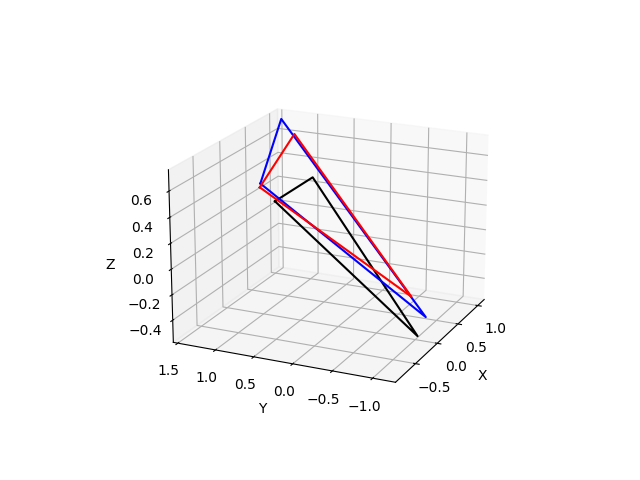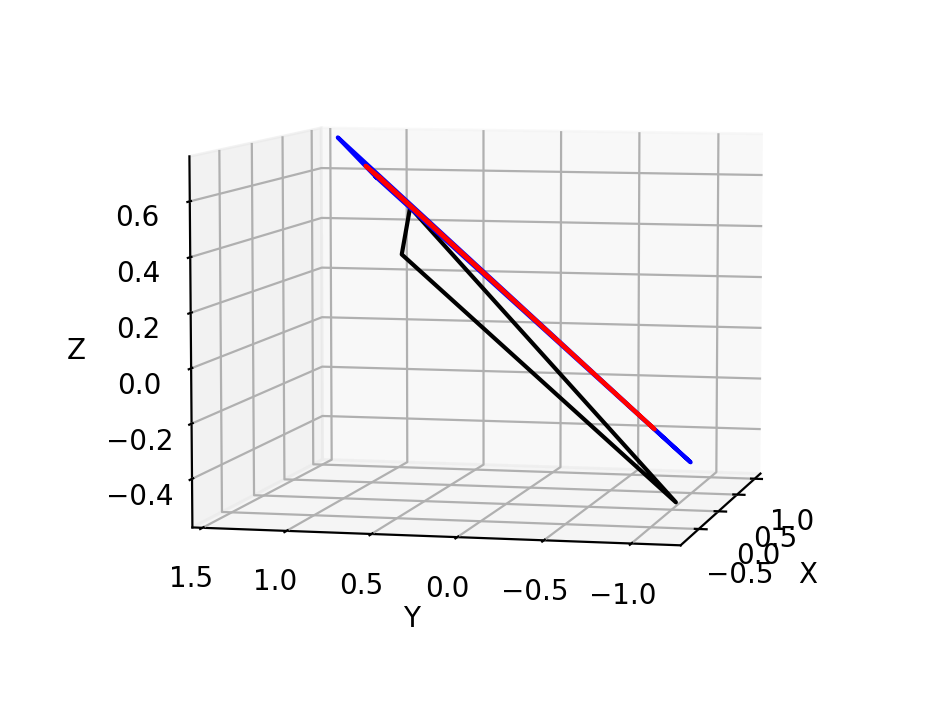# 速度更新公式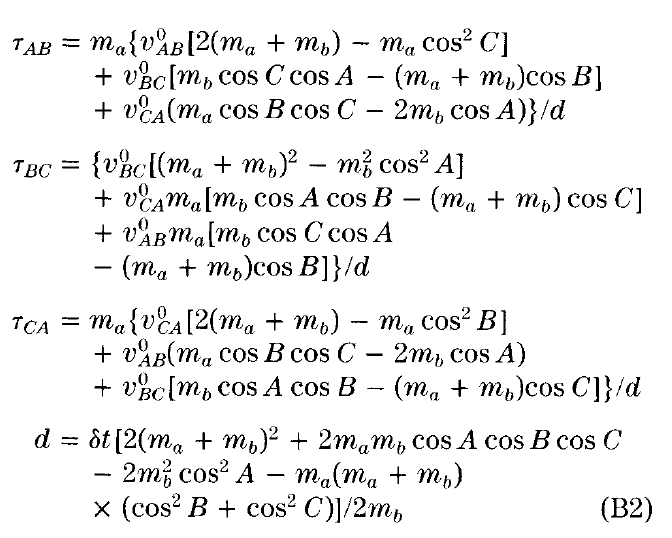# 参考文章

1. SETTLE: An Analytical Version of the SHAKE and RATTLE Algorithm for Rigid Water Models. Shuichi Miyamoto and Peter A.Kollman.

*注：本文所有的算法流程示意图，均来自于参考文章1

posted @ 2022-03-08 09:05  DECHIN  阅读(441)  评论(0编辑  收藏  举报